## Example Questions

1 3 Next →

### Example Question #21 : How To Factor An Equation

Factor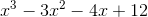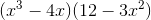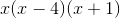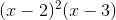cannot be factored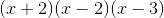Explanation:

This expression can be factored by grouping.

xcan be factored out of the first two terms.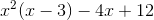-4 can be factored out of the second two terms.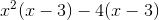Now we can factor out the term (x-3).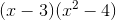x- 4 is a difference of squares. It can be factored into (x + 2)(x - 2).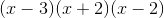1 3 Next →